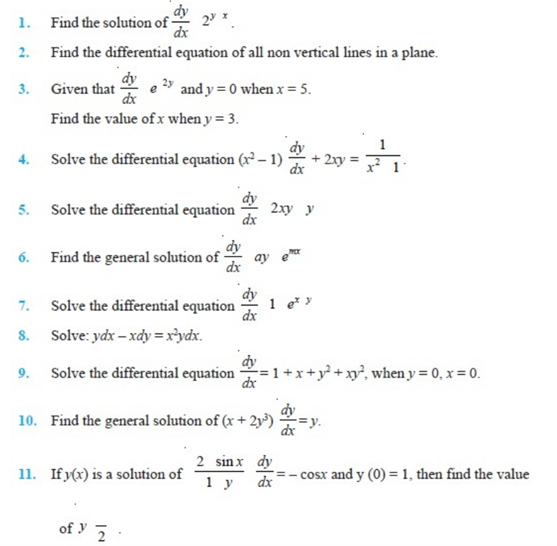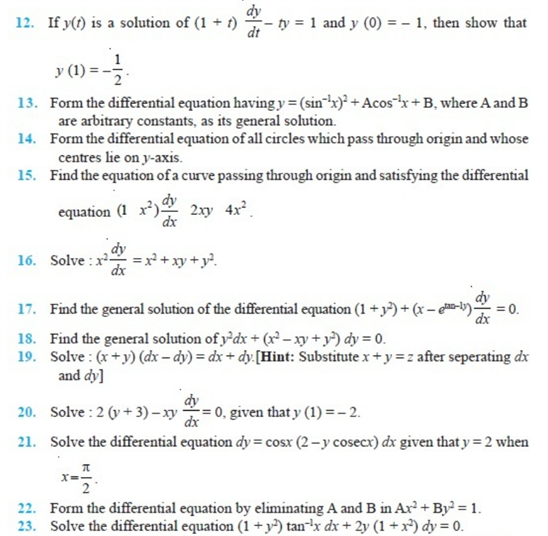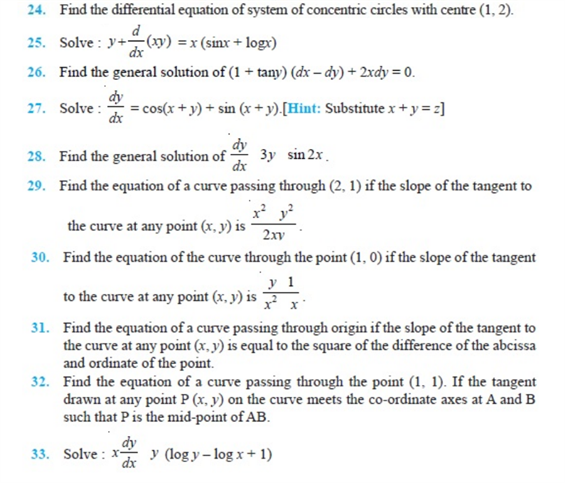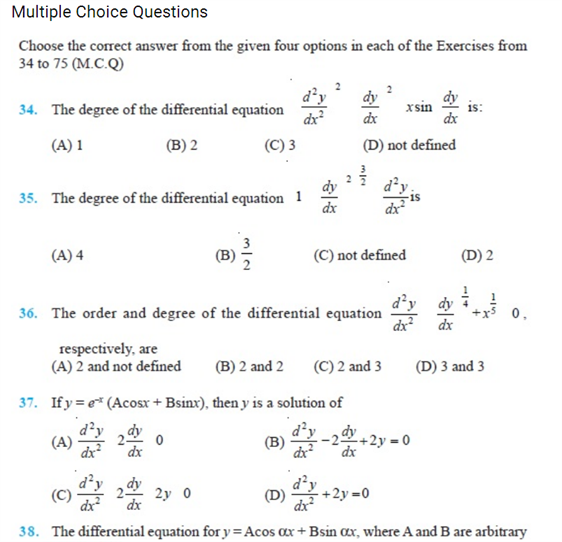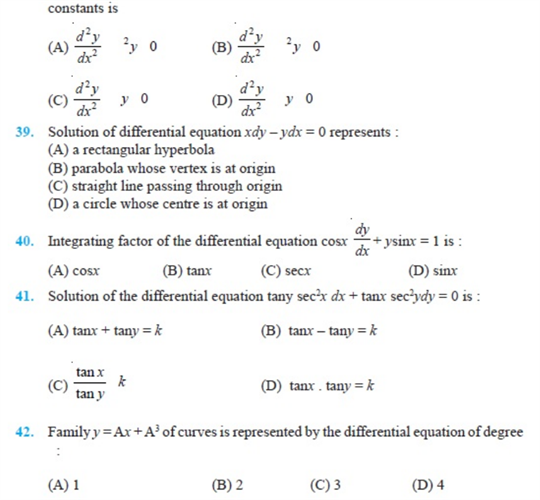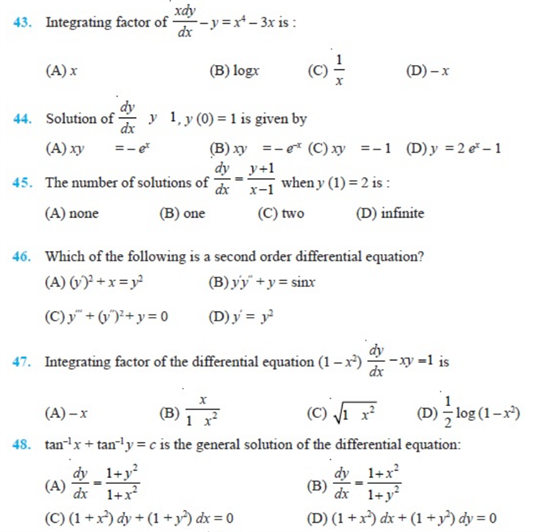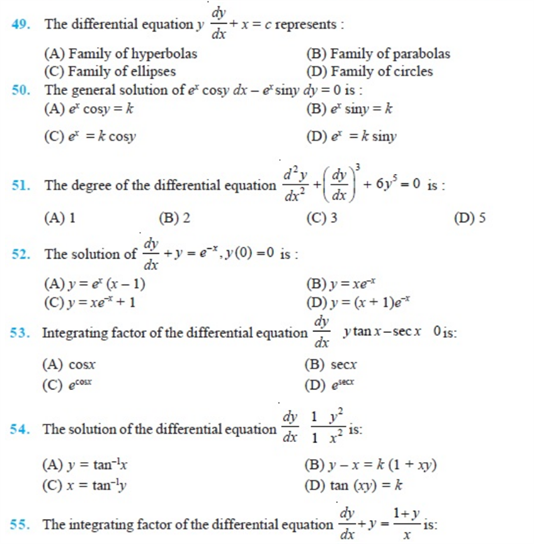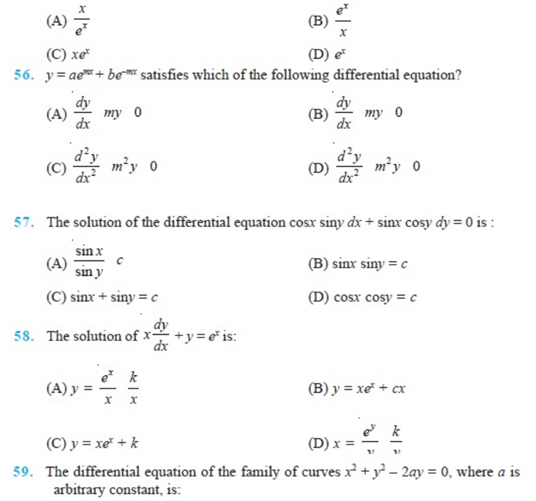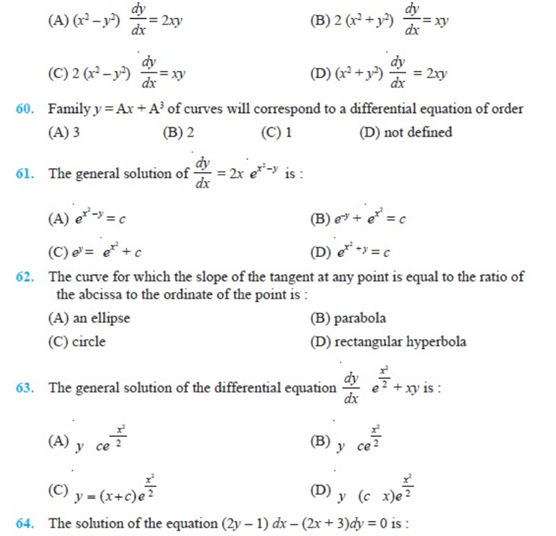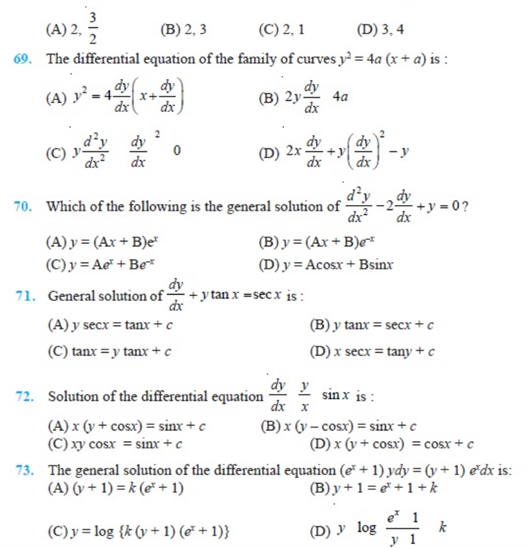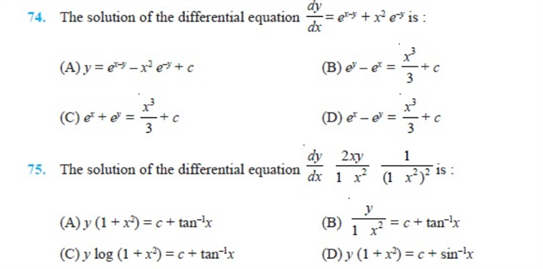# Chapter 9 - Differential Equations

## Important Questions For Class 12 Maths- Chapter 9- Differential Equations

Practical Geometry is one of the crucial topics of Maths chapter 9 of class 12. It is an important topic which also helps in preparing for Board exams for class 12(CBSE). Apart from studying and practising problems on differential equations from NCERT, students shall also practice these important questions.

Solving these important questions of class 12 maths chapter 9 will help you prepare for CBSE board exams too.

Also, check :

Differential Equation Formula

### Class 12 Chapter 9 Differential Equations

1. Solve dy/dx + y = 1.

2. Solve (x +1) dy/dx = 2xy

3. In a bank principal increases at the rate of 6% per year. In how many years Rs 2500 double itself.

4. Solve: dy/dx = cos(x+y)

5. Solve  the initial value problem: cos(a+b)dy =dx, y(0) = 0.

### Class 12 Chapter 9- Differential Equation NCERT Questions